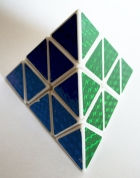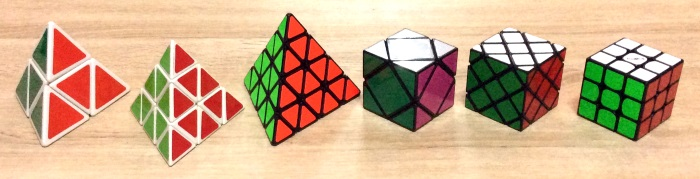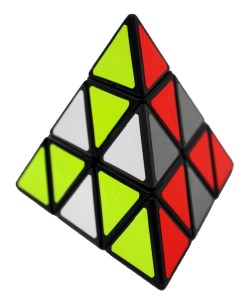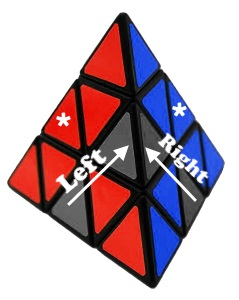# PyraminxThe Pyraminx, also known as the triangle Rubik's Cube is a tetrahedron-shaped 3-layered twisty puzzle, having four triangular faces which are all divided into nine identical smaller triangles. This is the second best selling puzzle toy in the World after the Rubik's Cube with over 100 million pieces sold.

It was invented by Uwe Mèffert (Germany) in 1971 but it was patented only later seeing the success of the Rubik's Cube in 1981. Meffert invented several other famous puzzles like the Megaminx, Skewb, Skewb Diamond, Teraminx, Dogic (icosahedron-shaped puzzle), and the list goes on. Other inventors were inspired by the 4-axle mechanism, Tony Fisher used it for the Golden Cube and Golden Egg.

The first official WCA Pyraminx competition was organized in 2003 and the winner was the American Andy Bellenir who solved the puzzle in 14.09 seconds. These days the best results range from 1 to 3 seconds, but the World record is under one second.

## The Pyraminx familyThe first puzzle on the image is not a 2x2 Pyraminx because that would be too simple where you would only have to turn the peaks and that would solve it instantly. The Pyramorphix is actually a 2x2 shape mod.
The third one is a Master Pyraminx, the 4x4 version of the puzzle.
It might be hard to believe that the Skewb is a Pyraminx shape mod. If you take apart the two puzzles you can see that they're both built on a similar core mechanism.

## How to solve a Pyraminx

Solving a Pyraminx is much easier than a Rubik's Cube. It has 3,732,480 possible permutations which is relatively a small number (similar to the 2x2x2 Pocket Cube), so a personal computer can easily go through all of the possible scrambles. It can be solved from any random position in less than 12 moves. I'm presenting below the easiest solution where you only have to learn a couple algorithms.

### 1. NotationLet's mark the four corners of the puzzle with letters, just like in the notation of the Rubik's Cube, a letter by itself means a clockwise 120o turn of the corner and an apostrophe marks a counterclockwise turn (eg. R'). We are going to use these letters to describe algorithms in this tutorial. For example: L' F L F'

We can easily notice that there are three tipes of pieces: Corner tips (A), centers having three stickers (B) and edges with two stickers (C).

### 2. Solving the tips and centersThe solution of the Pyraminx begins with turning the 4 corners (A) to match the center pieces. Tthis is just a simple turn for each corner because the three sides of the center pieces (B) are linked together. When the tips are OK, deep turn the puzzle to make the three center colors match on all faces. Try to reach the state on the image above! When you're done all that's left is solving the remaining 6 edge pieces (C).

### 3. Two edges next to one cornerIn this stage we want to solve three edges around the top corner. Use the Right and Left algorithms below depending on the direction of the insertion. These leave the pieces marked with the asterisk (*) in place. Usually you'll need an algorithm for the last piece because the first two can be solved intuitively.

Right algorithm: R F R' F'
Left algorithm: L' F' L F

### 4. Last layer edges

All that's left is to permute/cycle the last layer edges. Take the puzzle in your hands with the scrambled face on the left and perform the requred turns, depending on your case.Clockwise cycle: U' F U F'
Counterclockwise cycle: U L' U' L
Flip tow edges: F U' F' U F' L F L'